# How to Calculate and Solve for Turbine Stiffness | Irrigation Water Requirement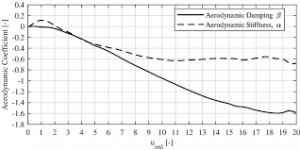The image above represents turbine stiffness.

To compute for turbine stiffness, three essential parameters are needed and these parameters are Modulus of Elasticity for Tubing (E), Moment of Inertia of Tubing (I) and Mean Radius of Tubing (r).

The formula for calculating turbine stiffness:

σ = EI /

Where:

σ = Turbine Stiffness
E = Modulus of Elasticity for Tubing
I = Moment of Inertia of Tubing
r = Mean Radius of Tubing

Let’s solve an example;
Find the turbine stiffness when the modulus of elasticity for tubing is 12, the moment of inertia of tubing is 10 and the mean radius of tubing is 4.

This implies that;

E = Modulus of Elasticity for Tubing = 12
I = Moment of Inertia of Tubing = 10
r = Mean Radius of Tubing = 4

σ = EI /
σ = (12)(10) / (4)³
σ = 120 / 64
σ = 1.875

Therefore, the turbine stiffness is 1.875.

Calculating for the Modulus of Elasticity for Tubing when the Turbine Stiffness, the Moment of Inertia of Tubing and the Mean Radius of Tubing is Given.

E = σ x r³ / I

Where;

E = Modulus of Elasticity for Tubing
σ = Turbine Stiffness
I = Moment of Inertia of Tubing
r = Mean Radius of Tubing

Let’s solve an example;
Find the modulus of elasticity for tubing when the turbine stiffness is 20, the moment of inertia of tubing is 10 and the mean radius of tubing is 2.

This implies that;

σ = Turbine Stiffness = 20
I = Moment of Inertia of Tubing = 10
r = Mean Radius of Tubing = 2

E = σ x r³ / I
E = 20 x 2³ / 10
E = 20 x 8 / 10
E = 160 / 10
E = 16

Therefore, the modulus of elasticity for tubing is 16.

Calculating for the Moment of Inertia of Tubing when the Turbine Stiffness, the Modulus of Elasticity for Tubing and the Mean Radius of Tubing is Given.

I = σ x r³ / E

Where;

I = Moment of Inertia of Tubing
σ = Turbine Stiffness
E = Modulus of Elasticity for Tubing
r = Mean Radius of Tubing

Let’s solve an example;
Find the moment of inertia of tubing when the turbine stiffness is 24, the modulus of elasticity for tubing is 14 and the mean radius of tubing is 4.

This implies that;

σ = Turbine Stiffness = 24
E = Modulus of Elasticity for Tubing = 14
r = Mean Radius of Tubing = 4

I = σ x r³ / E
I = 24 x 4³ / 14
I = 24 x 64 / 14
I = 1536 / 14
I = 109.7

Therefore, the moment of inertia of tubing is 109.7.

Calculating for the Mean Radius of Tubing when the Turbine Stiffness, the Moment of Inertia of Tubing and the Modulus of Elasticity for Tubing is Given.

r = 3√(EI / σ)

Where;

r = Mean Radius of Tubing
σ = Turbine Stiffness
E = Modulus of Elasticity for Tubing
I = Moment of Inertia of Tubing

Let’s solve an example;
Find the mean radius of tubing when the turbine stiffness is 18, the modulus of elasticity is 28 and the moment of inertia of tubing is 10

This implies that;

σ = Turbine Stiffness = 18
E = Modulus of Elasticity for Tubing = 28
I = Moment of Inertia of Tubing = 10

r = 3√(EI / σ)
r = 3√((28)(10) / 18)
r = 3√(280 / 18)
r = 3√15.55
r = 2.49

Therefore, the mean radius of tubing is 2.49.

Nickzom Calculator – The Calculator Encyclopedia is capable of calculating the turbine stiffness.

To get the answer and workings of the turbine stiffness using the Nickzom Calculator – The Calculator Encyclopedia. First, you need to obtain the app.

You can get this app via any of these means:

To get access to the professional version via web, you need to register and subscribe for NGN 2,000 per annum to have utter access to all functionalities.
You can also try the demo version via https://www.nickzom.org/calculator

Apple (Paid) – https://itunes.apple.com/us/app/nickzom-calculator/id1331162702?mt=8
Once, you have obtained the calculator encyclopedia app, proceed to the Calculator Map, then click on Agricultural under Engineering.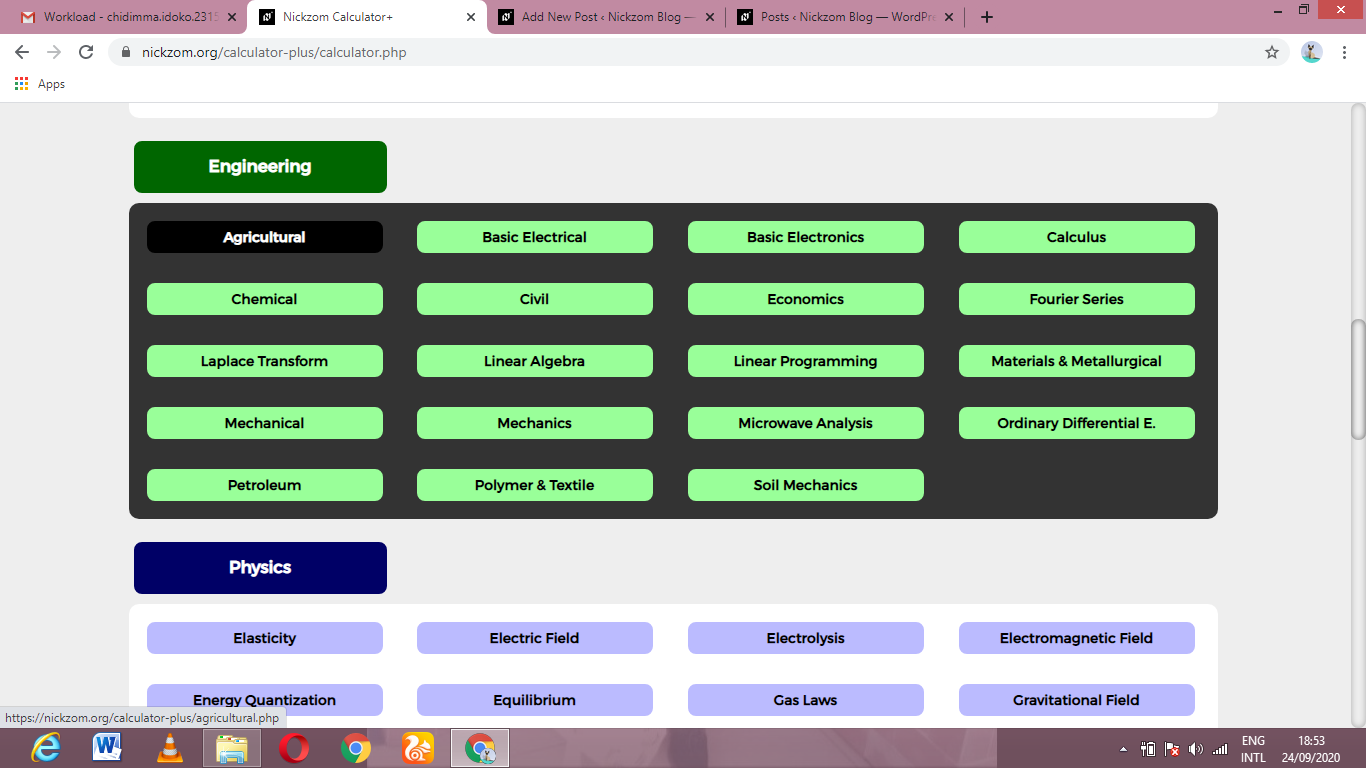Now, Click on Irrigation Water Requirement under AgriculturalNow, Click on Turbine Stiffness under Irrigation Water Requirement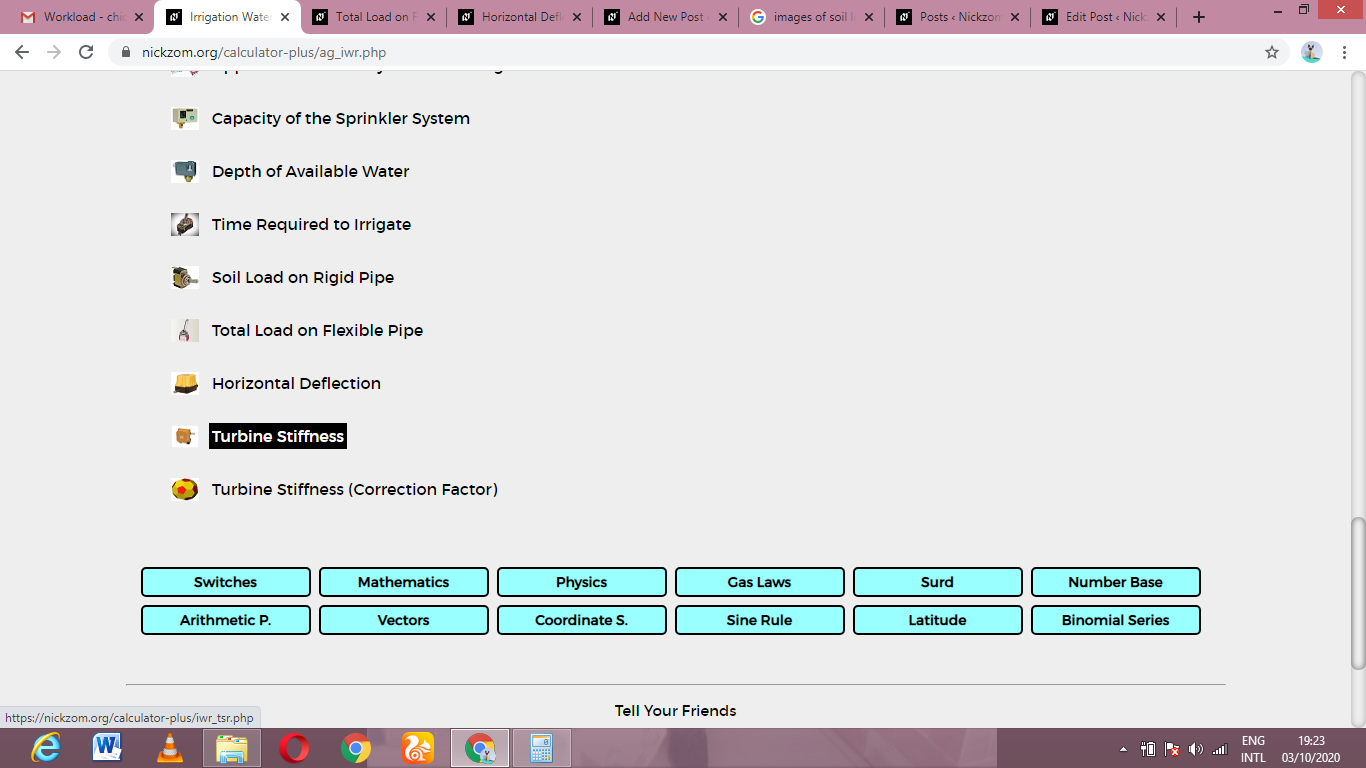The screenshot below displays the page or activity to enter your values, to get the answer for the turbine stiffness according to the respective parameters which is the Modulus of Elasticity for Tubing (E), Moment of Inertia of Tubing (I) and Mean Radius of Tubing (r).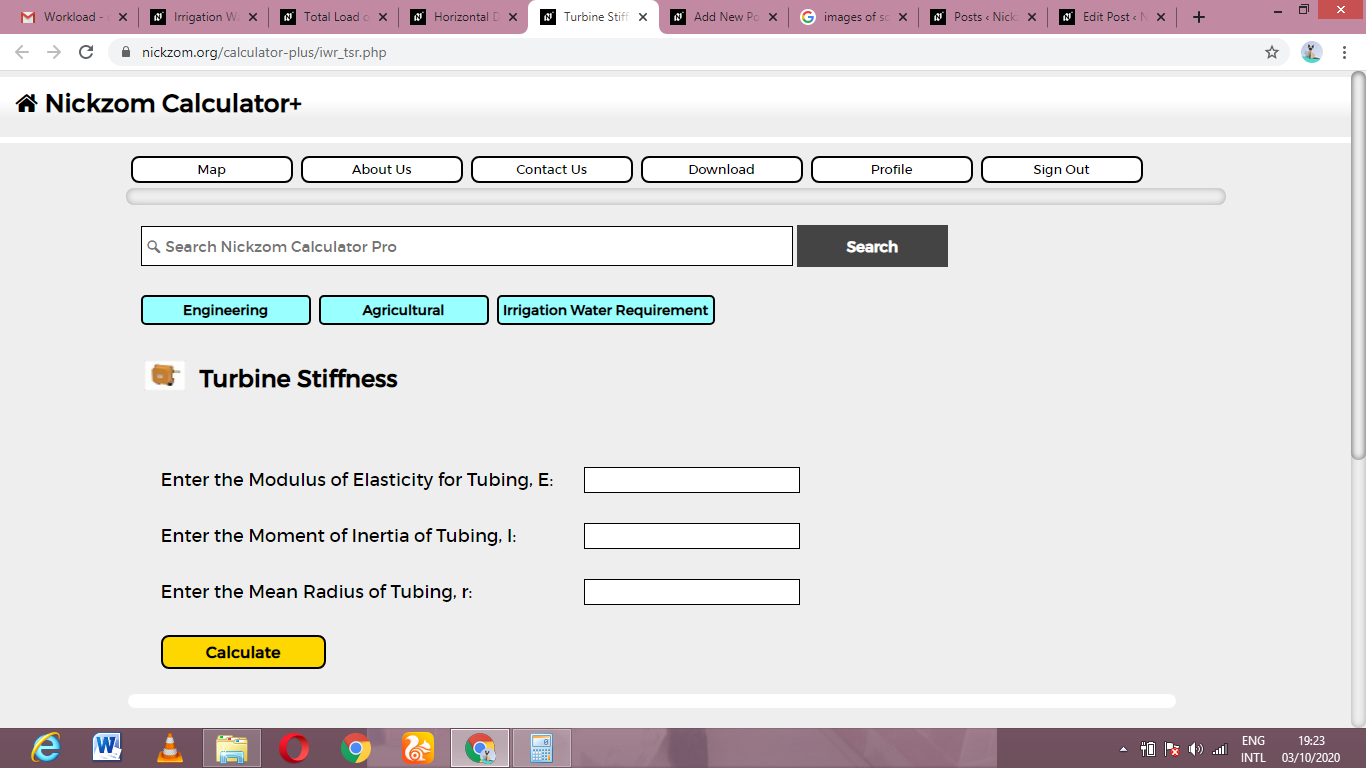Now, enter the values appropriately and accordingly for the parameters as required by the Modulus of Elasticity for Tubing (E) is 12, Moment of Inertia of Tubing (I) is 10 and Mean Radius of Tubing (r) is 4.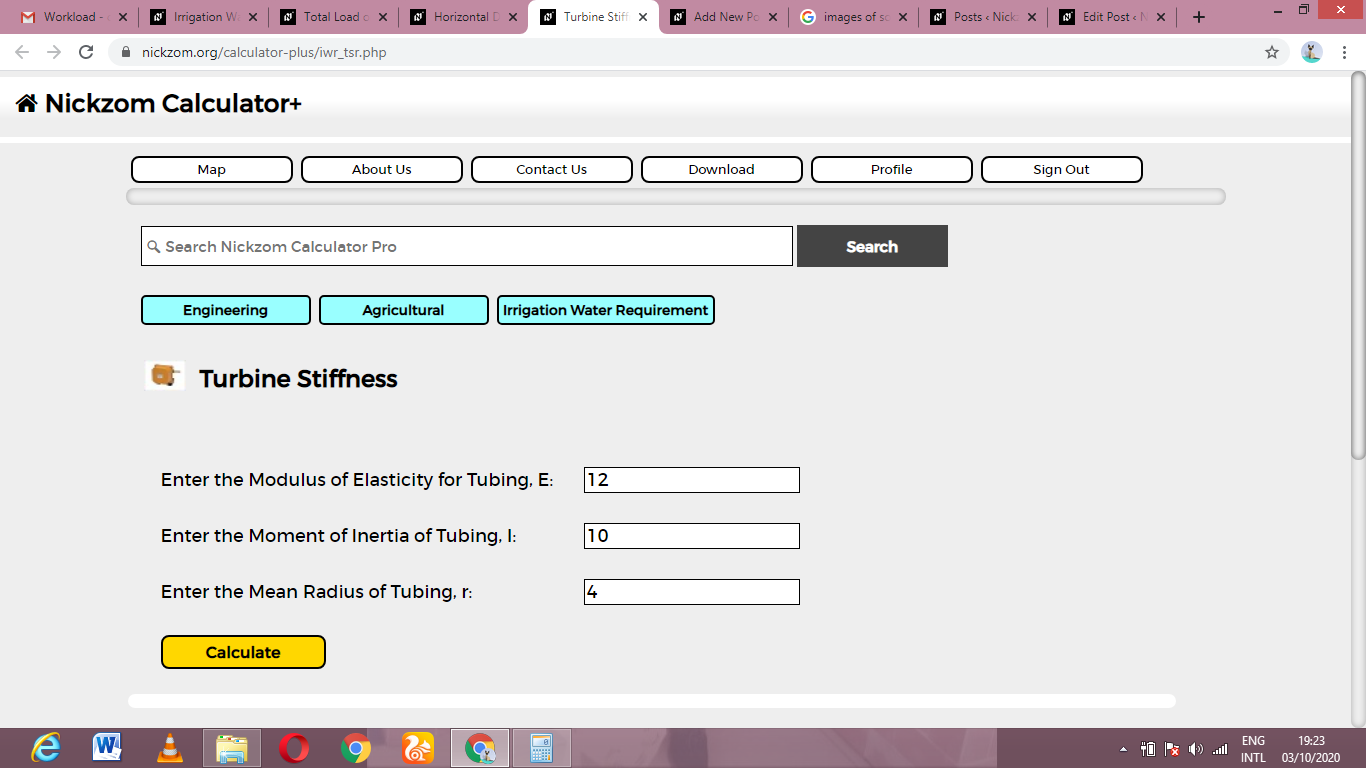Finally, Click on Calculate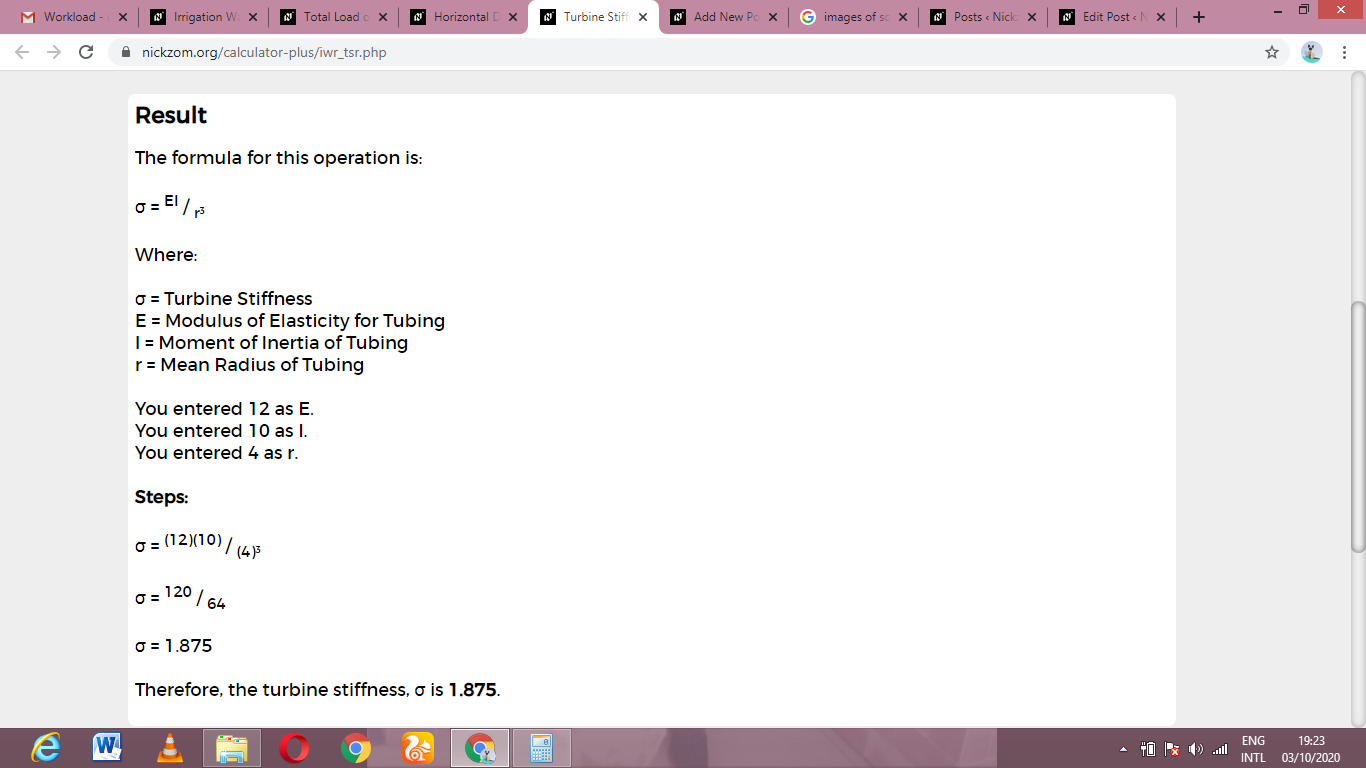As you can see from the screenshot above, Nickzom Calculator– The Calculator Encyclopedia solves for the turbine stiffness and presents the formula, workings and steps too.